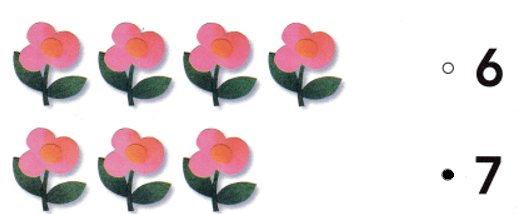# Texas Go Math Kindergarten Lesson 6.1 Answer Key Count and Order to 10

Refer to our Texas Go Math Kindergarten Answer Key Pdf to score good marks in the exams. Test yourself by practicing the problems from Texas Go Math Kindergarten Lesson 6.1 Answer Key Count and Order to 10.

## Texas Go Math Kindergarten Lesson 6.1 Answer Key Count and Order to 10

Explore

DIRECTIONS: Point to the numbers in the top workspace as you count forward to 10. Trace and write the numbers in order in the bottom workspace as you count forward to 10.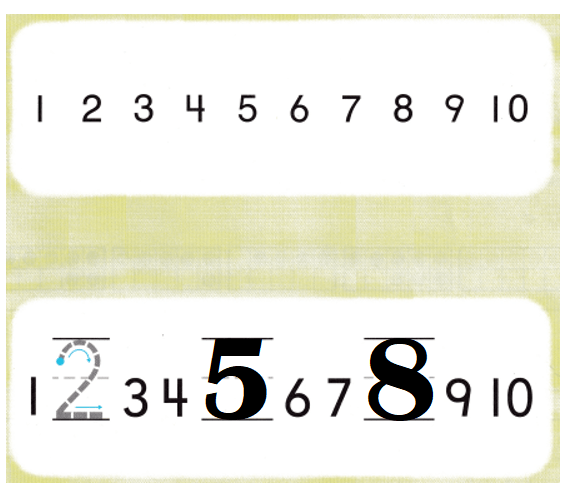Explanation:
Numbers in order from 1 to 10:
1, 2, 3, 4, 5, 6, 7, 8, 9, 10.

Share and Show
DIRECTIONS: 1-2. Count the dots of each color in the ten frames. Write the numbers. Look at the next line. Write the numbers in order as you count forward starting from the dashed number.

Question 1.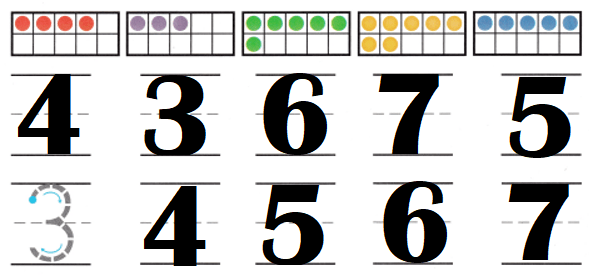Explanation:
Number of dots in the frames:
4, 3, 6, 7, 5.
Numbers in order:
3, 4, 5, 6, 7.

Question 2.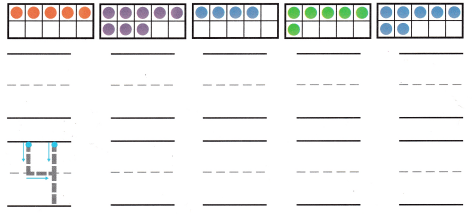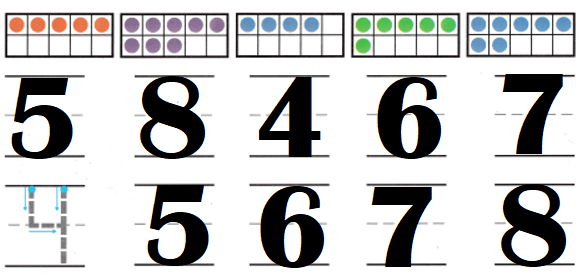Explanation:
Number of dots in the frames:
5, 8, 4, 6, 7.
Numbers in order:
4, 5, 6, 7, 8.

DIRECTIONS: 3-4. Count the dots of each color in the ten frames. Write the numbers. On the next line, write the numbers in order as you count forward starting from the dashed number.

Question 3.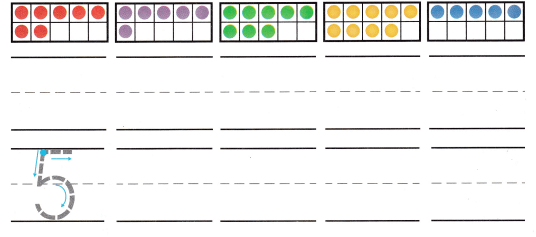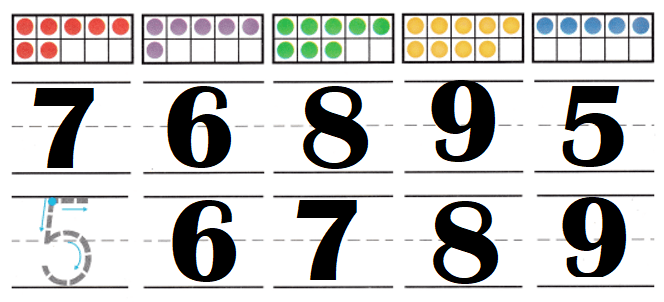Explanation:
Number of dots in the frames:
7, 6, 8, 9, 5.
Numbers in order:
5, 6, 7, 8, 9.

Question 4.Explanation:
Number of dots in the frames:
9, 7, 10, 6, 8.
Numbers in order:
6, 7, 8, 9, 10.

HOME ACTIVITY Write the numbers 1 to 10 in order on a piece of paper. Ask your child to point to each number as he or she counts to 10. Repeat beginning with a number other than 1 when counting.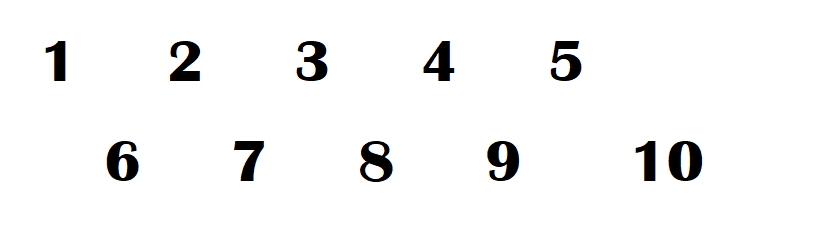Explanation:
I wrote number from 1 to 10. My son read all number from 1 to 10. He read all from 2 to 10.

DIRECTIONS: 5. Kate listed the numbers 5 through 10 in order. Look at the numbers. Write the numbers that are missing from Kate’s list. 6. Choose the correct answer. How many footprints are there?

Problem Solving
Question 5.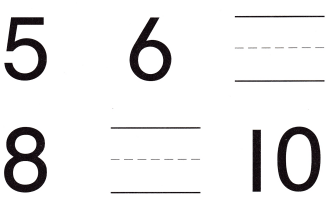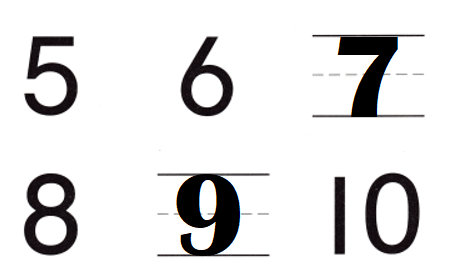Explanation:
Numbers:
5, 6, 7, 8, 9, 10.

Question 6.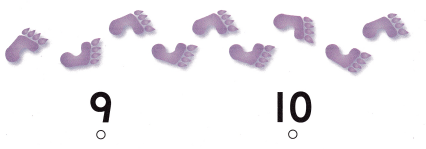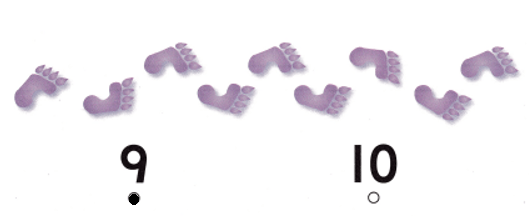Explanation:
Number of foot prints:
9.

### Texas Go Math Kindergarten Lesson 6.1 Homework and Practice Answer Key

DIRECTIONS: 1-2. Count the dots of each color in the ten frames. Write the numbers. On the next line, write the numbers in order as you count forward starling from the dashed number.

Question 1.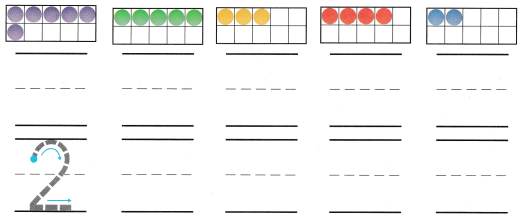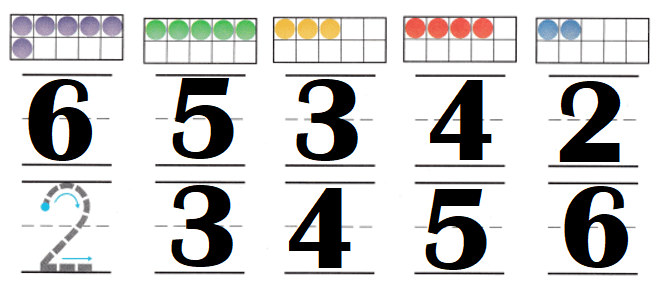Numbers of dots in the frames:
6, 5, 3, 4, 2.
Numbers in order:
2, 3, 4, 5, 6.

Explanation:

Question 2.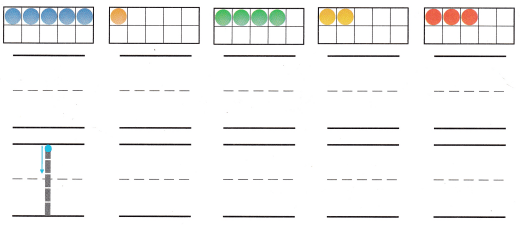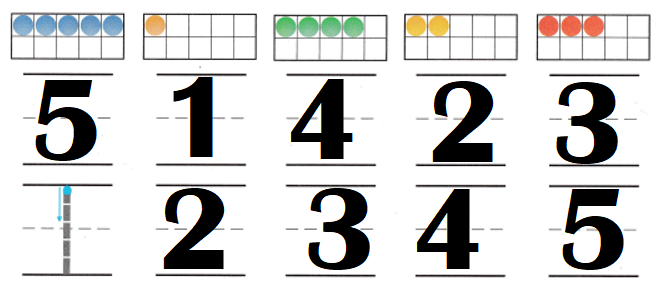Explanation:
Numbers of dots in the frames:
5, 1, 4, 2, 3.
Numbers in order:
1, 2, 3, 4, 5.

DIRECTIONS: Choose the correct answer. 3. How many balloons are there? 4. How many butterflies are there? 5. How many flowers are there?

Lesson Check
Question 3.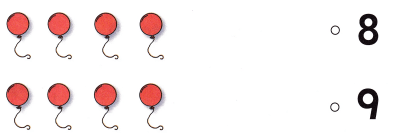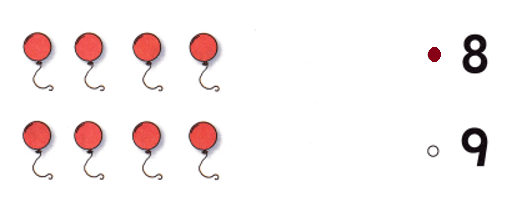Explanation:
Number of balloons = 8.

Question 4.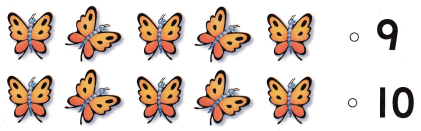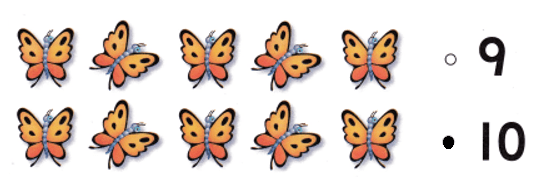Explanation:
Number of butterflies = 10.

Question 5.